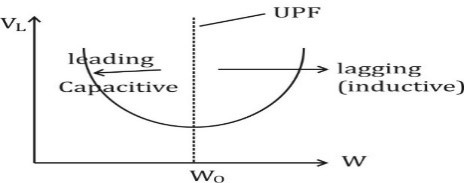Engineering Jobs   »   uprvunl je electrical quiz,

# UPRVUNL’21 EE: Daily Practices Quiz 26-July-2021

Each question carries 1 mark.
Negative marking: 1/4 mark
Total Questions: 06
Time: 08 min.

Q1. The law that explains the generation of heat due to flow of current through a conductor is
(a) Kelvin’s law
(b) Joule’s law
(c) Ohm’s law

Q2. A capacitor stores energy in
(a) An electric field
(b) A magnetic field
(c) Dielectric dipole
(d) An electromagnetic field

Q3. Slip of an induction motor ……………. when the rotor starts gaining speed.
(a) Increases
(b) Decreases
(c) Remains same
(d) Increase or decrease

Q4. Current chopping usually occurs in
(a) Oil circuit breaker
(b) Minimum OCB
(c) MCB
(d) Air blast circuit breaker

Q5. At a frequency less than the resonant frequency_____:
(a) series circuit is capacitive and parallel circuit is inductive
(b) series circuit is inductive and parallel circuit is capacitive
(c) both circuits are inductive
(d) both circuits are capacitive

Q6. For induction motors at low values of slip:
(a) The torque slip is approximately a hyperbola
(b) The torque slip is approximately a straight line
(c) The torque slip curve is at its maximum
(d) The torque slip is approximately a parabola

SOLUTIONS

S1. Ans.(b)
Sol. Joule’s law:
The law explains that amount of heat production in a conductor is:
Directly proportional to the square of electric current flowing through it.
Is directly proportional to the resistance of the conductor.
Directly proportional to the time for which electric current flows through the conductor,
i.e., H=I^2 Rt

S2. Ans.(a)
Sol. A charged capacitor stores energy in the electrical field between its plates.
U_C=1/2 CV^2=1/2 QV

S3. Ans.(b)
Sol. Slip(s)=(N_s-N_r)/N_s
So, when the rotor starts gaining speed, due to increase of rotor speed, slip of an induction
motor decreases.

S4. Ans.(d)
Sol. Current chopping usually occurs in Air blast circuit breaker.
Current Chopping in circuit breaker is defined as a phenomenon in which current is forcibly interrupted before the natural current zero.

S5. Ans.(a)
Sol. For series Resonant RLC circuitFor series RLC circuit, nature of circuit below resonant frequency is capacitive and vice-versa for parallel RLC circuit.

S6. Ans.(b)
Sol. For induction motor –
T=(KS E₂²R₂)/(R₂²+(S×_2)²)
at low value of slip, S×_2≪R₂
⇒ T=(KSE₂²R₂)/(R₂²)
⇒ T∝S

Sharing is caring!